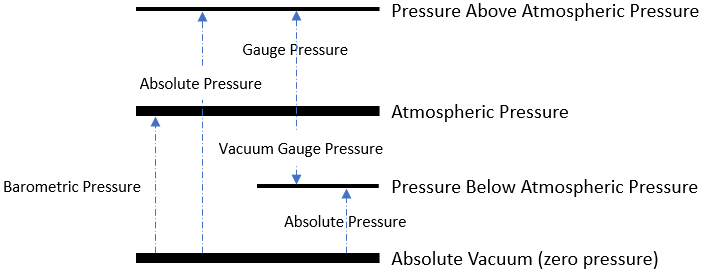## Absolute vs Gauge Pressure

Explain the differences between absolute, atmospheric, and gauge pressure. What about vacuum gauge pressure?

Hint
Pressure can be described as the force applied to an area:
$$P=\frac{F}{A}$$$Hint 2 Atmospheric pressure (barometric pressure) is the pressure within Earth’s atmosphere, which is defined as 101 kPa (or 14.7 psi). Pressure can be described as the force applied to an area: $$P=\frac{F}{A}$$$
Atmospheric pressure (barometric pressure) is the pressure within Earth’s atmosphere, which is defined as 101 kPa (or 14.7 psi). This standard atmosphere pressure unit (symbol: atm) is roughly equivalent to the average sea level atmospheric pressure on Earth, so the Earth’s atmospheric pressure at sea level is ~1 atm. The pressure relative to atmospheric pressure is called Gauge pressure. Gauge pressure is positive for pressures above atmospheric pressure, and negative for pressures below it. Negative gauge pressure is called vacuum gauge pressure. Absolute pressure is measured relative to the absolute zero pressure point, and is the sum of gauge pressure and atmospheric pressure:
$$P_{abs}=P_{atm}+P_{gauge}$$$$$P_{abs}=P_{atm}-P_{vacuum\:gauge}$$$
For example, if a car’s tire gauge reads 30 psi, then the absolute pressure is 30 psi + 14.7 psi = 44.7 psi.
As an overview:The simplest distinction between the gauge and absolute is that absolute pressure uses absolute zero as its standard baseline, while gauge pressure uses atmospheric pressure as its zero point.
Atmospheric pressure is the pressure within Earth’s atmosphere.